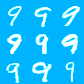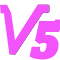[机器学习笔记#4] Neural Style 算法分析及caffe实验

2015 年德国的几位科研人员基于 google deep dream 的思路，提出了一种用 CNN自动生成带有特定艺术风格的图片的算法，发表了一篇名为A Neural Algorithm of Artistic Style的 tech notes， 可以说是 deep dream的美梦+艺术版本。

由于效果实在是太神奇，从那时起开始出现了很多利用此技术来美化用户图片的App和在线应用，比如手机应用 Prisma 和ostagram，以及deepart

楼主在读过这篇 tech notes后，被其想法的巧妙所折服，再加上目前网络上开源的版本已经有了基于 Torch, Theano 和 Tensorflow 的，但尚无 caffe版本。 于是产生了用 caffe来重复原文实验的想法。

该文的一个基本思路是：一张图片的内容（content）和风格（style）是可分离和重组的。于是我们可以用图片A的内容，加上图片B的风格，组合出一张新的图片，使之同时具有二者的内容和风格。

而这里CNN的作用在于，当用CNN识别一张图片时，当图片数据沿着各 convolution layer前向(forward)传播时，其包含的信息将越来越趋于精简，图片中的细节部分，或者说基础纹理和边缘，将逐渐淡去，而这些正是所谓“艺术风格”的体现之处，大概相当于平常说的“笔触”吧；而代表主干内容的结构信息，则会越来越清晰。这是CNN对人类视觉中“分层理解”的工作方式的一种近似模仿。

所以实际中，CNN中的浅层 convolution layer，主要对细节纹理信息进行提取，而这些 layer经过 activation layer 之后的输出，经过处理后，表征了一幅图片的 style 信息；而较深层次的convolution layer，则表征了图片的 content 信息。

具体的实现思路如下：

1. Find Content

基于上述思路，文中首先定义了用于度量一副图片在某一特定 layer $$l$$ 上 content 的特征 $$F^l$$ ，这个特征直接是选取了CNN在该 layer 后 activation 的输出的 feature map。 假设我们有一张图片 p，使我们在 layer $$l$$ 上得到了一个特征 $$P^l$$ ， 那么根据内容可分离的观点，这世界上一定存在另一张图片 x，其在同一CNN同一 layer上输出的特征 $$F^l$$ 无限接近于 $$P^l$$。也即是说图片 px 拥有相同的 content 。这里我们可以定义如下的 content loss function。
$$L_{content}(p,x,l)=\frac{1}{2} \sum_{ij} (F^l_{ij}-P^l_{ij})^2$$

解决的方法也非常鬼畜： 首先对 x 生成一张白噪声图片，作为初始值，然后用 gradient descent 和 backpropagation，在每一次迭代循环中计算 $$L_{content}(p,x,l)$$ 对 x每一个像素的导数，作为 x 该次 gradient descent 的 update。经过一定的循环次数后，x便可逐渐收敛为我们想要的目标图片。 由于在 backpropagation 中我们相当于使用了 $$L_{content}(p,x,l)$$作为 output layer，所以相对应的求导过程也需要我们给出。不过这里的 least-square loss 求导非常简单，初中生都会算（分段是因为 VGG使用 ReLu作为 activation layer的关系）：
$$\frac{\partial L_{content} }{\partial F^l_{ij}}= \begin{cases} ( F^l_{ij}- P^l_{ij} ) & if & F^l_{ij}>0\\\\ 0 & if & F^l_{ij}<0 \end{cases}$$ （实际上我本人比较想把这里的 least-square loss改成理论上更科学的 cross-entropy loss看看效果）
基于上述理论，楼主编写了如下基于 caffe的实验代码，待我一行行分析：

这一段是设置 caffe相关的环境，为省事直接copy了之前 deep dream的部分。

<code class="language-python"># imports and basic notebook setup
from cStringIO import StringIO
import numpy as np
import scipy.ndimage as nd
import PIL.Image
from IPython.display import clear_output, Image, display
import matplotlib.pyplot as plt
from numpy import linalg as LA
%matplotlib inline
import caffe

# If your GPU supports CUDA and Caffe was built with CUDA support,
# uncomment the following to run Caffe operations on the GPU.
caffe.set_mode_gpu()
caffe.set_device(0) # select GPU device if multiple devices exist

def showarray(a, fmt='jpeg'):
a = np.uint8(np.clip(a, 0, 255))
f = StringIO()
PIL.Image.fromarray(a).save(f, fmt)
display(Image(data=f.getvalue()))
</code>

根据原文，导入已经 train好的 VGG19模型

<code class="language-python">model_path = '/home/lzhang57/DeepLearning/caffe/models/bvlc_vgg19/' # substitute your path here
net_fn   = model_path + 'VGG_ILSVRC_19_layers_deploy.prototxt'
param_fn = model_path + 'VGG_ILSVRC_19_layers.caffemodel'

# Patching model to be able to compute gradients.
# Note that you can also manually add "force_backward: true" line to "deploy.prototxt".
model = caffe.io.caffe_pb2.NetParameter()
model.force_backward = True
open('tmp.prototxt', 'w').write(str(model))

net = caffe.Classifier('tmp.prototxt', param_fn,
mean = np.float32([104.0, 116.0, 122.0]), # ImageNet mean, training set dependent
channel_swap = (2,1,0)) # the reference model has channels in BGR order instead of RGB

# a couple of utility functions for converting to and from Caffe's input image layout
def preprocess(net, img):
return np.float32(np.rollaxis(img, 2)[::-1]) - net.transformer.mean['data']
def deprocess(net, img):
return np.dstack((img + net.transformer.mean['data'])[::-1])
</code>

读取 content 图片，一只萌猫。并指定用于计算 content feature的参考 layer，这里先设置成 'conv4_2'

<code class="language-python">img = np.float32(PIL.Image.open('data/cat.jpg'))
[h,w,c]=img.shape
content_img=preprocess(net, img)
### set stop layer ###
end='conv4_2'
src = net.blobs['data']
src.reshape(1,3,h,w)
dst = net.blobs[end]
### generate content image ###
src.data=content_img
net.forward(end=end)
Content=np.copy(dst.data)
</code>

<code class="language-python">### generate style image
target=np.float32(np.random.rand(h,w,3)*255)
target_img=preprocess(net, target)
step=2
src.data=target_img
### optimize target image
Loss=[];
max_iter=500
for iter in range(max_iter):
## compute feature
net.forward(end=end)
Feature=dst.data
tmp=Feature[:]-Content[:];
Loss.append(0.5*LA.norm(Feature-Content))
if( iter>0 and np.abs((Loss[-2]-Loss[-1])/Loss[-2])</code>

最后得到的输出图片如下，现在看来还平淡无奇，因为这里只是实现了“生成一张跟参考图片具有相同内容图片”的功能：
迭代过程中的 loss function 收敛情况如下：

在我研究 machine learning以前，如果有人告诉我可以像这样把一张白噪声图片只靠做减法，一点一点撸出一只猫来，我是打死也不信的。

今天就做这么多吧，实际上这里只实现了全部功能的 1/3，明天继续，睡觉zzz

更新计划：明天七夕，后天再继续

[修改于 4 年前 - 2016-08-09 12:19:59]

来自：计算机科学 / 机器学习

想参与大家的讨论？现在就 登录 或者 注册Cirno进士 专家 学者 笔友
文章
33
回复
358
学术分
2
2012/09/03注册，3 天前活动

Machine Learning, computer vision enthusiast

作者最新文章
相似文章推荐
%7B%22isDisplay%22%3Atrue%7D

仅供内部学术交流或培训使用，请先保存到本地。本内容不代表科创观点，未经原作者同意，请勿转载。

插入资源
全部
图片
视频
音频
附件
全部
未使用
已使用
正在上传
空空如也~
上传中..{{f.progress}}%
处理中..
上传失败，点击重试
等待中...
{{f.name}}
空空如也~
(视频){{r.oname}}
{{selectedResourcesId.indexOf(r.rid) + 1}}
处理中..
处理失败
插入表情
我的表情
共享表情
Emoji
上传
##### 注意事项
最大尺寸100px，超过会被压缩。为保证效果，建议上传前自行处理。
建议上传自己DIY的表情，严禁上传侵权内容。
点击重试等待上传{{s.progress}}%处理中...已上传
空空如也~
草稿箱
加载中...
此处只插入正文，如果要使用草稿中的其余内容，请点击继续创作。
{{fromNow(d.toc)}}
{{getDraftInfo(d)}}
标题：{{d.t}}
内容：{{d.c}}
{{forum.displayName}}